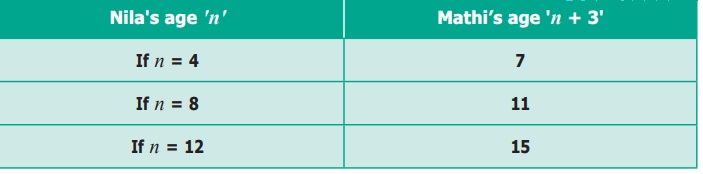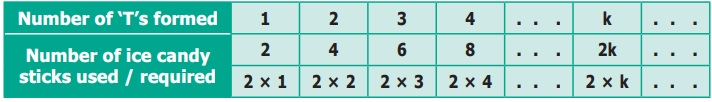Home | | Maths 6th Std | Understanding operations on Variables

# Understanding operations on Variables

Consider the following situations.

Understanding operations on Variables

Consider the following situations.

Situation 1:

Mathi is 3 years elder than his sister Nila. If we know Nila's age, can we find Mathi’s?If Nila’s age is ‘n’, you can see that Mathi’s age is always n + 3’. This is the advantage of using variables. We do not need different statements for different values of the age! As we give different values for ‘n’, we get different values for n + 3’. This is clear from the following table.

Situation 2:

Patterns using Ice Candy SticksPari and Manimegalai made some patterns with ice candy sticks.

To make one ‘T’, how many ice candy sticks are used by them? (Two sticks)

To make two ‘T’s, how many ice candy sticks are used by them? (Four sticks)

Continuing this, they prepared the following table to find the number of ice candy sticks used by themFrom the above table, it is clear that if the number of ‘T’s required by them is ‘k’ then the number of ice candy sticks required by them will be 2 × k = 2k. Here ‘k’ is a variable.

Tags : Introduction to Algebra | Term 1 Chapter 2 | 6th Maths , 6th Maths : Term 1 Unit 2 : Introduction To Algebra
Study Material, Lecturing Notes, Assignment, Reference, Wiki description explanation, brief detail
6th Maths : Term 1 Unit 2 : Introduction To Algebra : Understanding operations on Variables | Introduction to Algebra | Term 1 Chapter 2 | 6th Maths